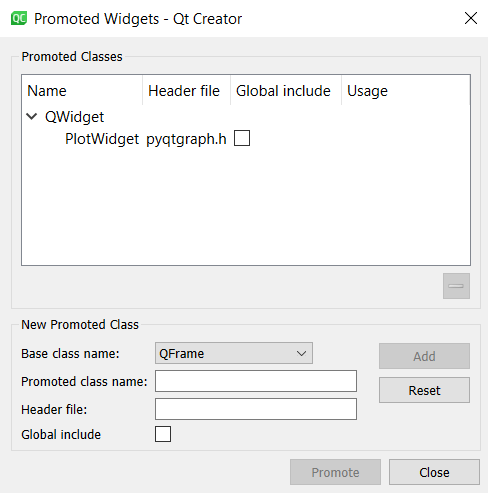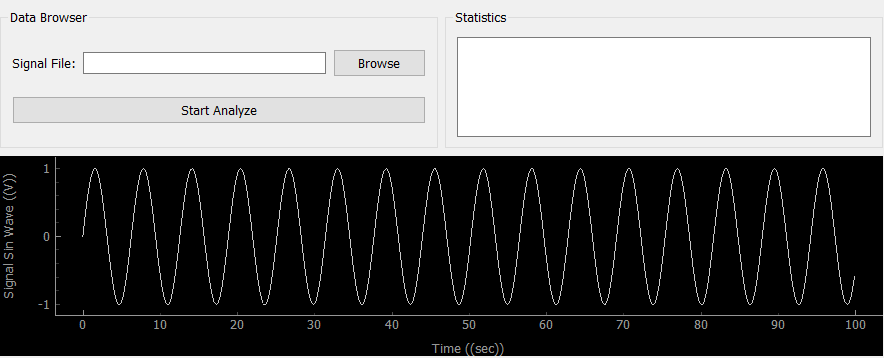# Add QT GUI to Python for plotting graphics

Qt framework (with QT Creator IDE) can be used to create a fancy interfaces for Python GUI application. Plotting graphics on a GUI is possible with pyqtgraph library.

Installing pyqtgraph –
There are several ways of installing pyqtgraph depending on your needs.

If you are using Anaconda you can install with:

```conda install -c anaconda pyqtgraph
```

Or with pip command:

```pip install pyqtgraph
```

Creation of plot widgets with QT Creator –

Add the buttons, text areas and other stuffs as usually done with QT Creator. To create a plot area you need to follow the steps:

1. Add widget to UI and give it a proper name like “widgetSignal”
2. Promote the widget to pyqtgraph1. In your python code call the UI you created with QT Creator.
2. Create a sin wave for plotting
3. Draw the graph on UI

 `from` `PyQt5 ``import` `QtWidgets, uic ` `import` `sys ` `import` `numpy as np ` ` `  `class` `MainWindow(QtWidgets.QMainWindow): ` `     `  `    ``def` `__init__(``self``, ``*``args, ``*``*``kwargs): ` `        ``super``(MainWindow, ``self``).__init__(``*``args, ``*``*``kwargs) ` `         ``# Load the UI Page ` `        ``self``. ui ``=` `uic.loadUi(``'mainwindow.ui'``, ``self``) ` `        ``# Create a sin wave ` `        ``x_time ``=` `np.arange(``0``, ``100``, ``0.1``); ` `        ``y_amplitude ``=` `np.sin(x_time) ` `         `  `        ``pltSignal ``=` `self``.widgetSignal ` `        ``pltSignal.clear() ` `        ``pltSignal.setLabel(``'left'``, ``'Signal Sin Wave'``, units ``=``'(V)'``) ` `        ``pltSignal.setLabel(``'bottom'``, ``'Time'``, units ``=``'(sec)'``) ` `        ``pltSignal.plot(x_time, y_amplitude, clear ``=` `True``) ` ` `  `        ``self``.ui.show() ` ` `  `def` `main(): ` `    ``app ``=` `QtWidgets.QApplication(sys.argv) ` `    ``window ``=` `MainWindow() ` `    ``sys.exit(app.exec_()) ` ` `  `if` `__name__ ``=``=` `'__main__'``: ` `    ``main()      `

Output:My Personal Notes arrow_drop_upIf you like GeeksforGeeks and would like to contribute, you can also write an article using contribute.geeksforgeeks.org or mail your article to contribute@geeksforgeeks.org. See your article appearing on the GeeksforGeeks main page and help other Geeks.

Please Improve this article if you find anything incorrect by clicking on the "Improve Article" button below.

Article Tags :

Be the First to upvote.

Please write to us at contribute@geeksforgeeks.org to report any issue with the above content.